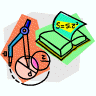## Mathematics, Department of## Faculty Publications, Department of Mathematics

#### Title

PERTURBATIONS OF CERTAIN REFLEXIVE ALGEBRAS

9-1994

#### Citation

Copyright 1994 Pacific Journal of Mathematics. Used by permission.

PACIFIC JOURNAL OF MATHEMATICS Vol. 165, No. 1, 1994 DOI: 10.2140/pjm.1994.165.161

#### Abstract

In this note we use cohomological techniques to prove that if there is a linear map between two CSL algebras which is close to the identity, then the two CSL algebras are similar. We use our result to show that if 2' is a purely atomic, hyperreflexive CSL with uniform infinite multiplicity which satisfies the 4-cycle interpolation condition, then there are constants d, C > 0 such that whenever L is another CSL such that d(Alg2' , AlgL) < d, then there is an invertible operator S such that S Alg2'S-1 = AlgL and IISII liS-III < 1 + Cd(AIg2' , AIgL).

COinS